Looking for VBA Tutorials?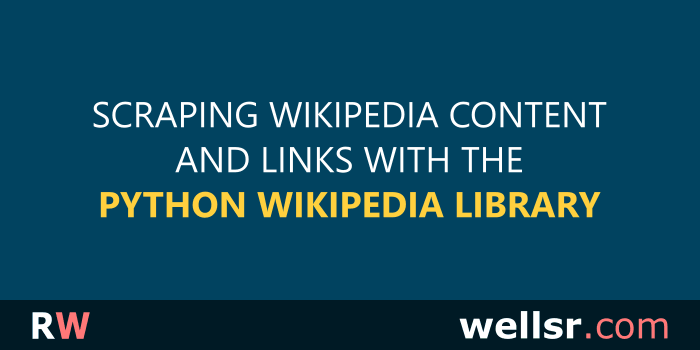Mar 20, 2020

This tutorials explains how to scrape Wikipedia pages using Python's Wikipedia library and extract information such as page names, links, images, and more.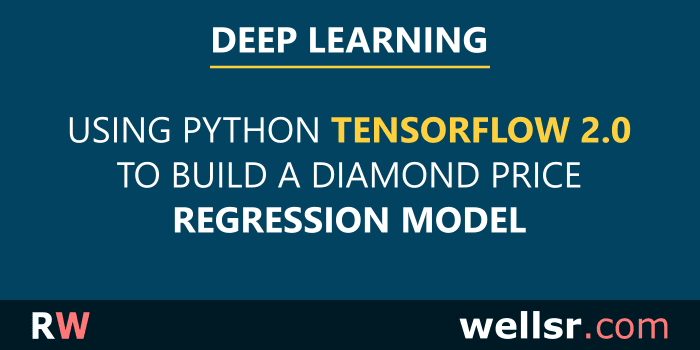Mar 13, 2020

This tutorial develops a diamond price prediction tool to explain how to perform regression tasks using Python's TensorFlow 2.0 library for deep learning.\$120.00 \$57.00

Want to learn more programming languages? We've combined each of our comprehensive VBA reference guides into a single bundle with over 200 tips and macros covering the 125 most important topics in VBA.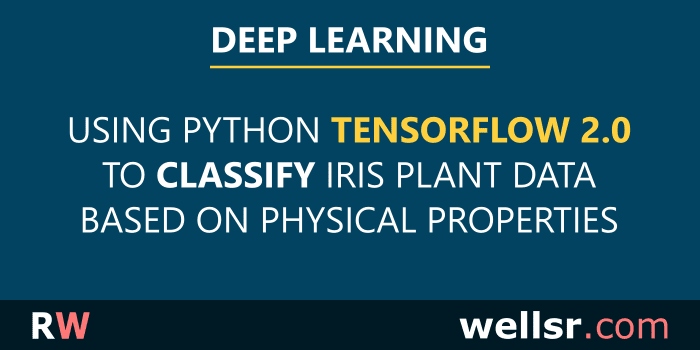Feb 23, 2020

This tutorial develops an Iris plant classification tool to explain how to perform classification tasks using Python's TensorFlow 2.0 library for deep learning.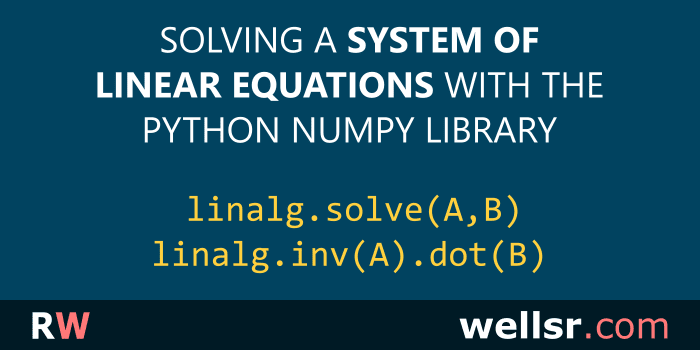Feb 7, 2020

This tutorial uses examples to explain how to solve a system of linear questions using Python's NumPy library and its linalg.solve and linalg.inv methods.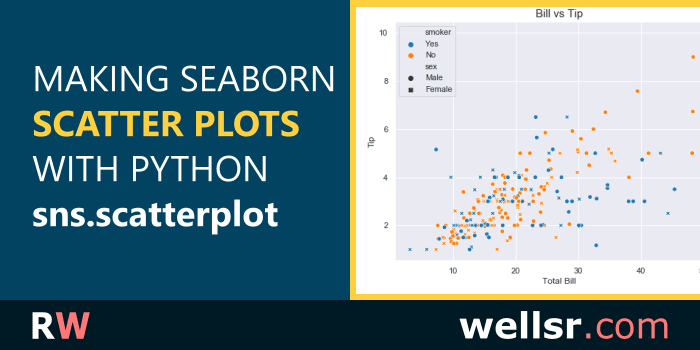Jan 17, 2020

The tutorial explains how to make different scatter plots using the Python Seaborn library. Several code examples demonstrate how to use sns.scatterplot.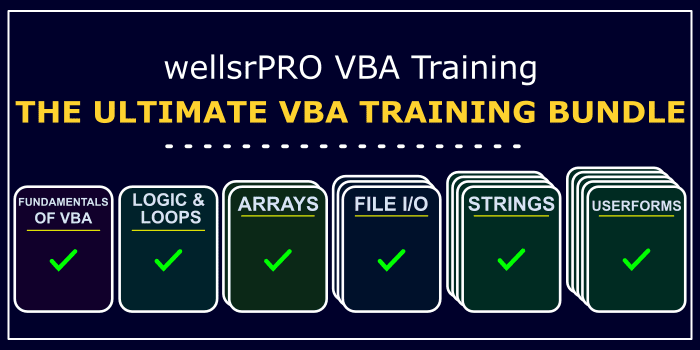\$120.00 \$57.00

We created a suite of 6 VBA cheat sheets with over 200 tips showing you everything you need to know to start making power Excel applications. Take a look!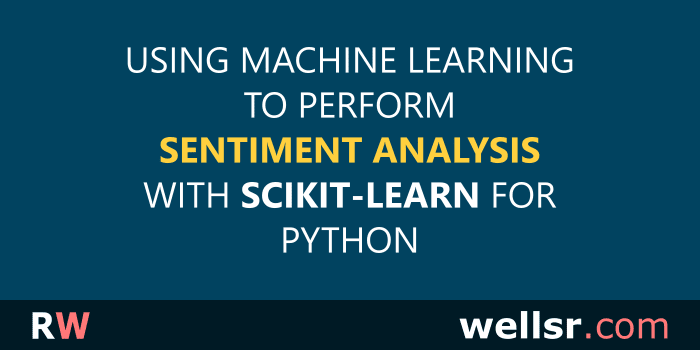Dec 20, 2019

This tutorial performs sentiment analysis using Python's Scikit-Learn library for machine learning. We use the sklearn library to analyze the sentiment of movie reviews.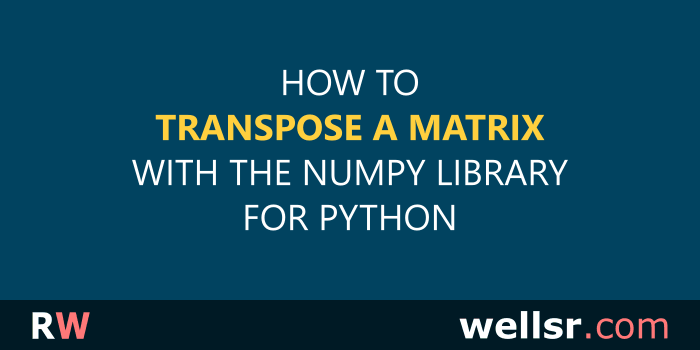Dec 13, 2019

This tutorial explains how to transpose a matrix using NumPy in Python and includes practical examples illustrating when you might need to transpose a matrix.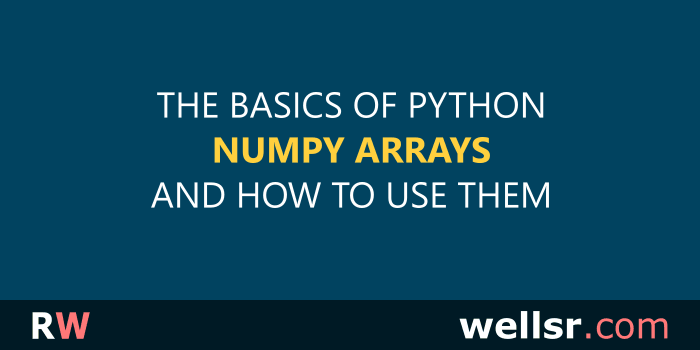Nov 27, 2019

This tutorial introduces the Python NumPy Library and explains how to use it to create arrays and perform arithmetic and matrix operations on NumPy arrays.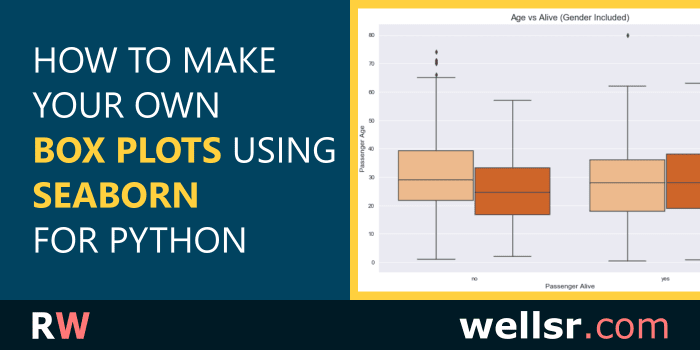Nov 22, 2019

This tutorial draws different Seaborn Boxplots using the Python Seaborn library. It includes examples for editing the colors, columns and labels of a box plot.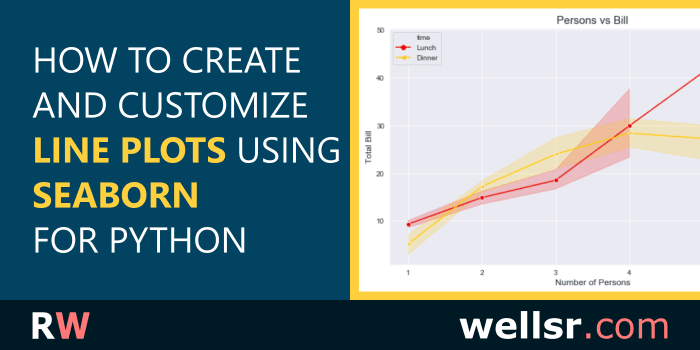Nov 12, 2019

This tutorial explains how to draw different line plots using the Python Seaborn library. It includes examples for setting line plot labels, markers and more.